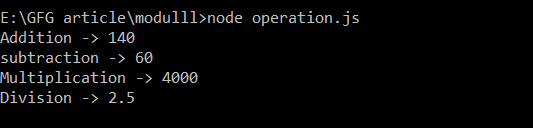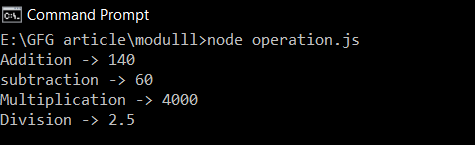Difference between module.exports and exports in Node.js

• Last Updated : 07 Oct, 2021

The module is a plain JavaScript Object representing the current module. It is local to each module and also it is private. It has exports property which is a plain JavaScript variable, set to module.exports. At the end of the file, Node.js return module.exports to the required function.

When we want to export a single class/variable/function from one module to another module, we use module.exports way.

Example: Create two file calculator.js and operation.js and export the Arithmetic class from calculator.js to operation.js using module.exports method. Here, we have created a class Arithmetic and exported the whole class using module.exports.

Filename: calculator.js

Javascript

 class Artimatics {    constructor(a, b) {        this.a = a;        this.b = b;    }      add() {        return this.a + this.b;    }    subtract() {        return this.a - this.b;    }      multiply() {        return this.a * this.b;    }      divide() {        if (this.b != 0) {            return this.a / this.b;        }        return "divided by zero !!!!";    }};  module.exports = Artimatics;

Filename: operation.js

Javascript

 const Artimatics = require('./calculator.js');  const op = new Artimatics(100,40);  console.log(`Addition -> \${op.add()}`);console.log(`subtraction -> \${op.subtract()}`);console.log(`Multiplication -> \${op.multiply()}`);console.log(`Division -> \${op.divide()}`);

Run the operation.js file using the following command:

node operation.js

Output:Using module.exports

When we want to export multiple variables/functions from one module to another, we use exports.

Example: Create a two file calculator.js and operation.js and export multiple functions from calculator.js file.

Filename: calculator.js

Javascript

 exports.add = (a, b) => a + b;exports.subtract = (a, b) => a - b;exports.multiply = (a, b) => a * b;exports.divide = (a, b) => {    if (b != 0) {        return a / b;    }    return `Divided by zero !!!`;}

Filename: operation.js

Javascript

 const Artimatics = require('./calculator.js');  console.log(`Addition -> \${Artimatics.add(100,40)}`);console.log(`subtraction -> \${Artimatics.subtract(100,40)}`);console.log(`Multiplication -> \${Artimatics.multiply(100,40)}`);console.log(`Division -> \${Artimatics.divide(100,40)}`);

Run the operation.js file using the following command:

node operation.js

Output:Using exports

Key difference between module.exports and exports:

My Personal Notes arrow_drop_up